• 这是一篇介绍蓝桥杯板子方波N555模块的博客 我自己的理解是将定时器0设置为计数模式，定时器1设置为计时模式来定1S的时间，然后定时器0记录的次数就是频率。 怎么设置呢只需要将TMOD设置为TMOD=0x04即可 寄存器...
https://blog.csdn.net/qq_37429313/article/details/86929724

这是一篇介绍蓝桥杯板子方波N555模块的博客

我自己的理解是将定时器0设置为计数模式，定时器1设置为计时模式来定1S的时间，然后定时器0记录的次数就是频率。

怎么设置呢只需要将TMOD设置为TMOD=0x04即可

寄存器TMOD高四位是设置定时器1工作方式，低四位是设置定时器0工作方式

C/T位是设置C/T==0是计时模式，C/T==1是计数模式，所以TMOD=0x04；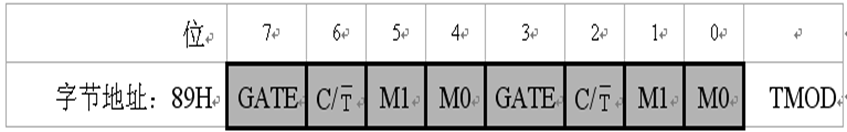之后是设置TH0和TL0位，此时可以设置为 TH0=TL0=0X00;也可以设置为 TH0=TL0=0XFF;

设置为TH0=TL0=0x00;就是用这两个记录次数。最后计算 num=(TH0<<8)+TL0;num就是1S内的次数

设置为TH0=TL0=0XFF;是用中断的方式计数，自己写中断函数，在中断函数里自己设置变量来计数。

工作时需要将P34和SIGNAL用调键帽连接在一起，这样P34才会有脉冲。

TH0=TL0=0x00;主要代码如下：

void Timer0Init(void)
{
AUXR |= 0x80;
TMOD = 0x04;    //方式1 16位模式；
TL0 = 0x00;        //设置定时初值
TH0 = 0x00;        //设置定时初值
TF0 = 0;        //清除TF0标志
TR0 = 1;        //定时器0开始计时
}
void Timer1Init(void)        //5毫秒@11.0592MHz
{
AUXR |= 0x40;        //定时器时钟1T模式
TMOD &= 0x0F;        //设置定时器模式
TL1 = 0x00;        //设置定时初值
TH1 = 0x28;        //设置定时初值
TF1 = 0;        //清除TF1标志
TR1 = 1;        //定时器1开始计时
EA=1;
ET1=1;
}
void main()
{
while(1)
{
if(T_flag)    //频率采集
{
T_flag=0;
num=(TH0<<8)+TL0;
TL0=0x00;
TH0=0x00;
TF0=0;
TR0=1;
}
}
}
void  time1() interrupt 3
{
static  uint T_cont=0;
T_cont++;
if(T_cont==200)
{     T_cont=0;
TF0 = 0;
TR0 = 0;
T_flag=1;
}
}

TH0=TL0=0xff;主要代码如下：

void Timer0() interrupt 1
{
sqre_count++;  //计数
}
while(1)
{
if(count_flag)
{
count_flag = 0;
sqre = sqre_count;   //sqre就是频率
sqre_count = 0;
TR0 = 1;
TR1 = 1;
}
}

上面那么多介绍的都N555下面介绍第十届的编程试题这里只放了框图，试题的话大家都能找到；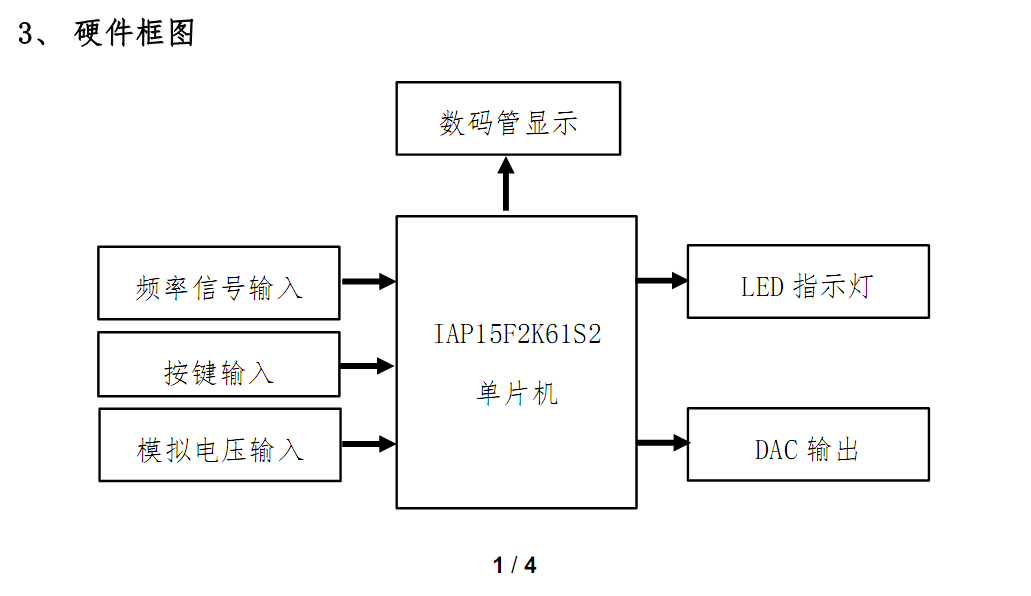我感觉这一届的试题比第九届的简单多了，按键部分也都是简单的逻辑但是因为没看方波所以直接就丢掉了一半的分数。具体实现的思路每个人都不一样，我就直接贴代码了；

主函数

#include<stc15F2K60S2.h>
#include<intrins.h>
#include<iic.h>
#define uchar unsigned char
#define uint  unsigned int
uchar tab[]={0xc0,0xf9,0xa4,0xb0,0x99,0x92,0x82,0xf8,0x80,0x90,0xbf,0xff,0xc1,0x8e};
uchar yi,er,san,si,wu,liu,qi,ba;
bit T_flag,set_flag,a_flag,L_flag,M_flag;
//T_flag 1S时间的标记 set_flag 电压和频率
//a_flag 电压固定 L_flag灯开关,M_flag数码管开关
void display31(uchar dat1,uchar dat2);
void keyscan();
void allinit();
void keyscan();
void delayms(uint ms);
void display1(uchar dat1,uchar dat2);
void display2(uchar dat1,uchar dat2);
void display3(uchar dat1,uchar dat2);
void display4(uchar dat1,uchar dat2);

void Timer0Init(void)		//1毫秒@11.0592MHz
{
AUXR |= 0x80;		//定时器时钟1T模式
TMOD = 0x05;
TL0 = 0x00;		//设置定时初值
TH0 = 0x00;		//设置定时初值
TF0 = 0;		//清除TF0标志
TR0 = 1;		//定时器0开始计时
}
void Timer1Init(void)		//5毫秒@11.0592MHz
{
AUXR |= 0x40;		//定时器时钟1T模式
TMOD &= 0x0F;		//设置定时器模式
TL1 = 0x00;		//设置定时初值
TH1 = 0x28;		//设置定时初值
TF1 = 0;		//清除TF1标志
TR1 = 1;		//定时器1开始计时
EA=1;
ET1=1;
}

void main()
{
allinit();
Timer0Init();
Timer1Init();
while(1)
{
keyscan();    //按键扫描
if(T_flag)    //频率采集
{
T_flag=0;
num=(TH0<<8)+TL0;
TL0=0x00;
TH0=0x00;
TF0=0;
TR0=1;
}
/*****电压*******************/
if(set_flag==0)
{
yi=12;er=11;san=11;si=11;wu=11;
if(a_flag==0)  //DAC固定2V
{
yi=12;er=11;san=11;si=11;wu=11;
liu=2;qi=0;ba=0;
}
if(L_flag==0)  //灯亮
{
{
if(a_flag==0)//固定
{
P2=0x80;P0=0xfe;
}
else if(a_flag==1)
{
P2=0x80;P0=0xee;
}
}
else
{
if(a_flag==0)//固定
{
P2=0x80;P0=0xfe;
}
else
{
P2=0x80;P0=0xea;
}
}
}
else
{
P2=0x80;P0=0xff;
}
}
/*******频率**********************/
else
{
yi=13;er=11;san=11;si=num/10000;wu=num/1000%10;
liu=num/100%10;qi=num/10%10;ba=num%10;
if(si==0)
{
si=11;
if(wu==0)
{
wu=11;
if(liu==0)  //数码管
{
liu=11;
}
}
}
if(L_flag==0)
{
if((num<1000)||(num>=5000&&num<10000))
{
P2=0x80;P0=0xfd;
}
else
{
P2=0x80;P0=0xf5;
}
}
else
{
P2=0x80;P0=0xff;
}
}
if(M_flag)//关闭所有数码管
{
yi=11;er=11;san=11;si=11;wu=11;
liu=11;qi=11;ba=11;
}
display1(yi,er);
display2(san,si);
if(set_flag==0&& M_flag==0)
display3(wu,liu);  //带小数点的
else if(set_flag==1)
display31(wu,liu); //不带小数点的
display4(qi,ba);

}
}
/****按键****************/
void keyscan()
{
if(P33==0)
{
delayms(10);
if(P33==0)
{
set_flag = ~set_flag;
}
while(!P33);
}
if(P32==0)
{
delayms(10);
if(P32==0)
{
a_flag = ~a_flag;
}
while(!P32);
}
if(P31==0)
{
delayms(10);
if(P31==0)
{
L_flag = ~L_flag;
}
while(!P31);
}
if(P30==0)
{
delayms(10);
if(P30==0)
{
M_flag = ~M_flag;
}
while(!P30);
}
}
//计时
void  time1() interrupt 3
{
static  uint T_cont=0;
T_cont++;
if(T_cont==200)
{	 T_cont=0;
TF0 = 0;
TR0 = 0;
T_flag=1;
}
}
{
uchar temp;
IIC_Start();
IIC_SendByte(0x90);
IIC_WaitAck();
IIC_WaitAck();
IIC_Stop();

IIC_Start();
IIC_SendByte(0x91);
IIC_WaitAck();
temp=IIC_RecByte();
IIC_Stop();
return temp;
}
/******初始化*********************/
void allinit()
{
P2=0xa0;P0=0x00;
P2=0x80;P0=0xff;
P2=0xc0;P0=0xff;
P2=0xff;P0=0xff;
}

void display1(uchar dat1,uchar dat2)
{
P2=0xc0;P0=0x01;
P2=0xff;P0=tab[dat1];
delayms(1);
P2=0xc0;P0=0x02;
P2=0xff;P0=tab[dat2];
delayms(1);
}
void display2(uchar dat1,uchar dat2)
{
P2=0xc0;P0=0x04;
P2=0xff;P0=tab[dat1];
delayms(1);
P2=0xc0;P0=0x08;
P2=0xff;P0=tab[dat2];
delayms(1);
}
void display3(uchar dat1,uchar dat2)
{
P2=0xc0;P0=0x10;
P2=0xff;P0=tab[dat1];
delayms(1);
P2=0xc0;P0=0x20;
P2=0xff;P0=tab[dat2]&0x7f;
delayms(1);
}
void display31(uchar dat1,uchar dat2)
{
P2=0xc0;P0=0x10;
P2=0xff;P0=tab[dat1];
delayms(1);
P2=0xc0;P0=0x20;
P2=0xff;P0=tab[dat2];
delayms(1);
}
void display4(uchar dat1,uchar dat2)
{
P2=0xc0;P0=0x40;
P2=0xff;P0=tab[dat1];
delayms(1);
P2=0xc0;P0=0x80;
P2=0xff;P0=tab[dat2];
delayms(1);
P2=0xc0;P0=0xff;
P2=0xff;P0=0xff;
}
void delayms(uint ms)
{
uint i,j;
for(i=0;i<ms;i++)
for(j=0;j<855;j++);
}

IIC 驱动：

/*
程序说明: IIC总线驱动程序
软件环境: Keil uVision 4.10
硬件环境: CT107单片机综合实训平台 8051，12MHz
日    期: 2011-8-9
*/

#include<stc15F2K60S2.h>
#include "intrins.h"

#define DELAY_TIME 20

//总线引脚定义
sbit SDA = P2^1;  /* 数据线 */
sbit SCL = P2^0;  /* 时钟线 */

void IIC_Delay(unsigned char i)
{
do{_nop_();}
while(i--);
}
//总线启动条件
void IIC_Start(void)
{
SDA = 1;
SCL = 1;
IIC_Delay(DELAY_TIME);
SDA = 0;
IIC_Delay(DELAY_TIME);
SCL = 0;
}

//总线停止条件
void IIC_Stop(void)
{
SDA = 0;
SCL = 1;
IIC_Delay(DELAY_TIME);
SDA = 1;
IIC_Delay(DELAY_TIME);
}

//发送应答
//void IIC_SendAck(bit ackbit)
//{
//    SCL = 0;
//    SDA = ackbit;  					// 0：应答，1：非应答
//    IIC_Delay(DELAY_TIME);
//    SCL = 1;
//    IIC_Delay(DELAY_TIME);
//    SCL = 0;
//    SDA = 1;
//    IIC_Delay(DELAY_TIME);
//}

//等待应答
bit IIC_WaitAck(void)
{
bit ackbit;

SCL  = 1;
IIC_Delay(DELAY_TIME);
ackbit = SDA;
SCL = 0;
IIC_Delay(DELAY_TIME);
return ackbit;
}

//通过I2C总线发送数据
void IIC_SendByte(unsigned char byt)
{
unsigned char i;

for(i=0; i<8; i++)
{
SCL  = 0;
IIC_Delay(DELAY_TIME);
if(byt & 0x80) SDA  = 1;
else SDA  = 0;
IIC_Delay(DELAY_TIME);
SCL = 1;
byt <<= 1;
IIC_Delay(DELAY_TIME);
}
SCL  = 0;
}

//从I2C总线上接收数据
unsigned char IIC_RecByte(void)
{
unsigned char i, da;
for(i=0; i<8; i++)
{
SCL = 1;
IIC_Delay(DELAY_TIME);
da <<= 1;
if(SDA) da |= 1;
SCL = 0;
IIC_Delay(DELAY_TIME);
}
return da;
}


自己给自己的话，这次比赛虽然不是什么大比赛，但也学会了很多，从过完年回来开始准备，2个星期把之前的省赛题做完，后边感觉自己会的挺多的了，后边就没看省赛之前没出过的模块，当时还做了官网模拟题就是把N555模块跳了过去。 今天之后这个比赛就算过去了，以后不管做什么事情都要要求自己做好最充分的准备，万万不可骄傲。


展开全文• 蓝桥杯单片机省赛全历年真题已解全历年国赛真题题目（史上最全） 省赛代码全为原创，持续更新中。 （其中部分代码为各处转载） 蓝桥杯单片机比赛加油！与君共勉！ 码云：https://gitee.com/quanshiyicunzai/events
• 蓝桥杯单片机历年真题，比较少，但是真东西，找了网上的资源综合的，没有图片，全是PDF和Word
• ## 蓝桥杯大赛单片机比赛的心得总结

千次阅读 多人点赞 2019-03-03 22:55:39
翻了下以前做过的一些项目和比赛，发现了之前准备比赛的一些注意事项和心得，分享给大家希望大家能够避免错误拿高分。 适当的延时很重要，可以解决一些不正常现象 ds1302读取的时间是BCD码,操作时间时换成10进制...
翻了下以前做过的一些项目和比赛，发现了之前准备比赛的一些注意事项和心得，分享给大家希望大家能够避免错误拿高分。

适当的延时很重要，可以解决一些不正常现象
ds1302读取的时间是BCD码,操作时间时换成10进制操作例：(shi / 16) * 10 + shi % 16
**********************************使用串口时*********************
每次只接受和发送一个字符，字符用单引号 ‘’ 字符串用双引号“”
if(SBUF == ‘a’）而不是if(SBUF == "a")

**1.总中断EA定时器中断ET0和串行通信中断ES记得开

**2.52单片机程序不可以直接使用P32的写法，15单片机可以，52使用前需要先进行定义P32=P3^2

*3.main函数下面不能忘记写while（1）{}

4.简单初始化函数必须写在while1（）{}上面，不能写在里面

6.写iic的读字节函数不用这行程序：temp=0.39*temp;

7.pwm的精度不同表现在占空比上，比如8位pwm占空比最小只能是1：256，尔10位pwm占空比可以使1：1024.

8.定时器溢出时间=周期/占空比

9.定时器不好用的时候可以用ds1302代替

10.ds1302和ds18b20部分子程序名相同，注意！！

11.串口通信发送或接受一个字节就进入中断，TI或RI = 1；使用串口助手时一定要选对波特率和文本模式或HEX模式

***12.修改时钟时，将BCD转换成10进制在进行加减，时到24变成0，分和秒到60变成0，修改时分秒显示时 /10  不是/16

13.超声波用的TR1,TF1,TH1,TL1.用的是定时器1,串口通信的波特率在模式1下与定时器1的溢出率有关

14.写数据到EEPROM时，地址最好0x00到0x56,最好间隔一个地址存多个数据，存入的数据最大为255，超过可把数据分开存储

15.读取时钟时先关中断在读，写EEPROM和读iic和温度时，加延时，注意++i和i++的区别使用

16.iic返回数都是unsigned char,adc_val / 255.0f * 5.ds18b20返回数是float，先读low，再读high函数里temp定义为int，temp*0.0625。读函数都要初始化两次，超声波distance = t * 0.017

18.当矩阵键盘和串口同时使用时，串口不要用串口1；P30端口会相互影响；

19.定义数组时预设内存比实际使用大一点
速查表：

char -128 ~ +127 (1 Byte)
short -32767 ~ + 32768 (2 Bytes)
unsigned short 0 ~ 65536 (2 Bytes)
int -2147483648 ~ +2147483647 (4 Bytes)
unsigned int 0 ~ 4294967295 (4 Bytes)
long == int
long long -9223372036854775808 ~ +9223372036854775807 (8 Bytes)
double 1.7 * 10^308 (8 Bytes)

unsigned int 0～4294967295
long long的最大值：9223372036854775807
long long的最小值：-9223372036854775808
unsigned long long的最大值：1844674407370955161

__int64的最大值：9223372036854775807
__int64的最小值：-9223372036854775808
unsigned __int64的最大值：18446744073709551615


展开全文• 数码管显示几乎是每次蓝桥杯单片机设计与开发组竞赛的必考部分，相对于使用LCD1602作为显示设备，使用数码管来显示能够更好的考察参赛选手的单片机基本功，因为CT107D开发平台的设计使得数码管的显示不仅牵扯到...
数码管显示几乎是每次蓝桥杯单片机设计与开发组竞赛的必考部分，相对于使用LCD1602作为显示设备，使用数码管来显示能够更好的考察参赛选手的单片机基本功，因为CT107D开发平台的设计使得数码管的显示不仅牵扯到数码管显示的 基本内容，还涉及到74HC573锁存器，74HC02异或门,74HC138译码器，以及中断的知识，所以这是值得我们重视的一个部分。

一、原理分析
根据CT107D的原理图我们可以看出，数码管显示同样涉及到74HC573、74HC138和74HC02，这与上一节中的LED灯、继电器和蜂鸣器的控制大同小异。如下图，蓝色标注为位选控制端口，由锁存器U6来控制，需配置红色标注的Y6C来控制锁存；黄色标注为段选控制端口，由锁存器U7来控制，需配置红色标注的Y7C来控制锁存。而，控制Y6C与Y7C则需要通过74HC18和74HC02来进行控制，如下图，Y6C由Y6和WR共同控制，74HC02是一个4路2输入或非门功能，WR为低电平，要使Y6C为高，则Y6必须为低电平，即74HC138译码器的三个输入端应该为100，即P2^7=1,P2^6=0,P2^5=0。又只需要操作P2口的这三位，不需要配置其他的五位，所以P2端口应该配置为：P2 = ((P2&0x1f)|0xC0)。同理，控制Y7C时P2端口应该置为：P2 = ((P2&0x1f)|0xE0)。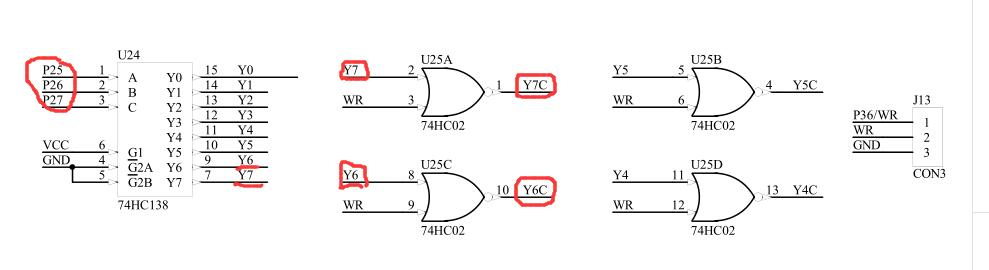二、示例程序
这里采用前后台的编程思想，即使用定时器中断来处理显示部分，作为前台，而逻辑处理与数据采集部分等则在主程序流程中来完成，作为后台。在下面这段程序中，定时器每隔2ms中断一次执行一次显示程序，然后继续执行主程序的任务，我们只要修改需要显示的数组即可实现显示不同的数值，使显示部分模块化，程序显示部分只提供外部接口（数组），不修改显示函数，这样不容易出错，而且能够使显示更方便。

#include "reg52.h"
#define uchar unsigned char
#define uint unsigned int

uchar code tab[] = {0xc0,0xf9,0xa4,0xb0,0x99,0x92,0x82,0xf8,0x80,0x90,0xff,0xbf};   //共阳极数码管段选编码数组
uchar dspbuf[] = {10,10,10,10,10,10,10,10};   //显示数据的数组
uchar dspcom = 0;   //位选控制变量

void display()  //显示函数
{
P2 = ((P2&0x1f)|0xE0);    //段选消隐
P0 = 0xff;
P2 &= 0x1f;

P2 = ((P2&0x1f)|0xC0);   //位选
P0 = 1<<dspcom;    //共阳极数码管位选
P2 &= 0x1f;

P2 = ((P2&0x1f)|0xE0);   //段选
P0 = tab[dspbuf[dspcom]];
P2 &= 0x1f;

if(++dspcom == 8){      //位选编码，循环显示8位
dspcom = 0;
}
}

void main()
{
TMOD = 0x01;   //定时器0工作方式1
TH0 = (65536-2000)/256;   //初始化
TL0 = (65536-2000)%256;
ET0 = 1;     //开定时器0中断允许位
EA = 1;      //开总中断
TR0 = 1;     //开定时器0

while(1)
{
dspbuf = 1;   //要显示不同的数据只需要修改此数组的值即可
dspbuf = 2;
dspbuf = 3;
dspbuf = 4;
dspbuf = 5;
dspbuf = 6;
dspbuf = 7;
dspbuf = 8;
}
}

void timer0() interrupt 1   //定时器0中断服务函数
{
TH0 = (65536-2000)/256;
TL0 = (65536-2000)%256;
display();   //2ms显示一次
}
展开全文• 个人觉得本题主要考的是定时器，本题如果直接用数码管延时扫描的话会很棘手，之前数码管显示函数我一般都是先位选后段选接着在后面延时个1毫秒，现在这题目用这种方式写好像不太行，正因为不行，我卡在这地方太久了...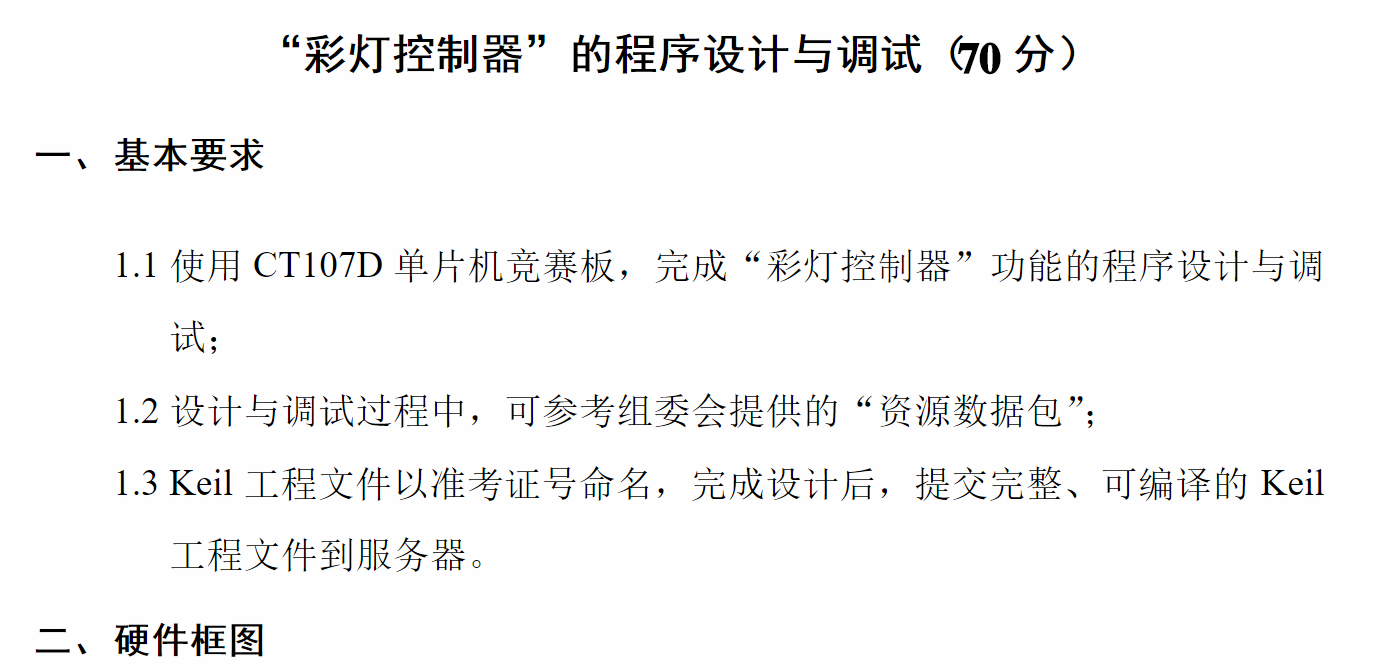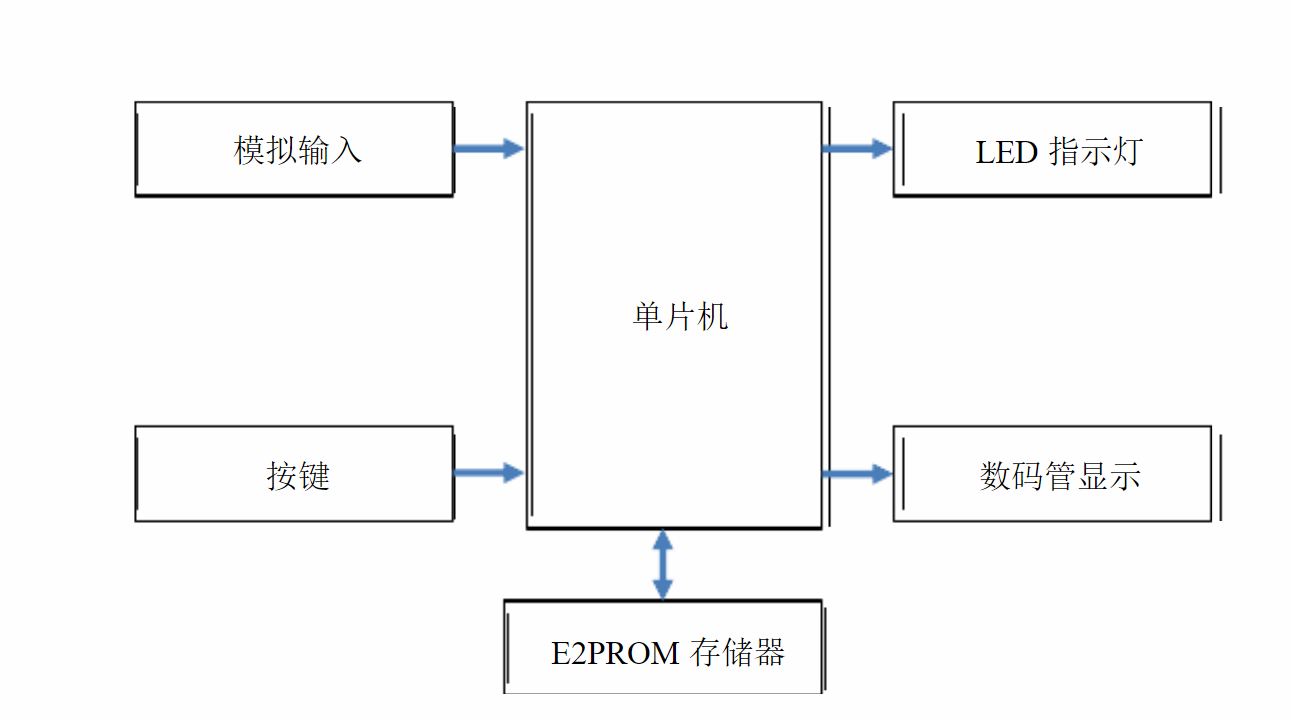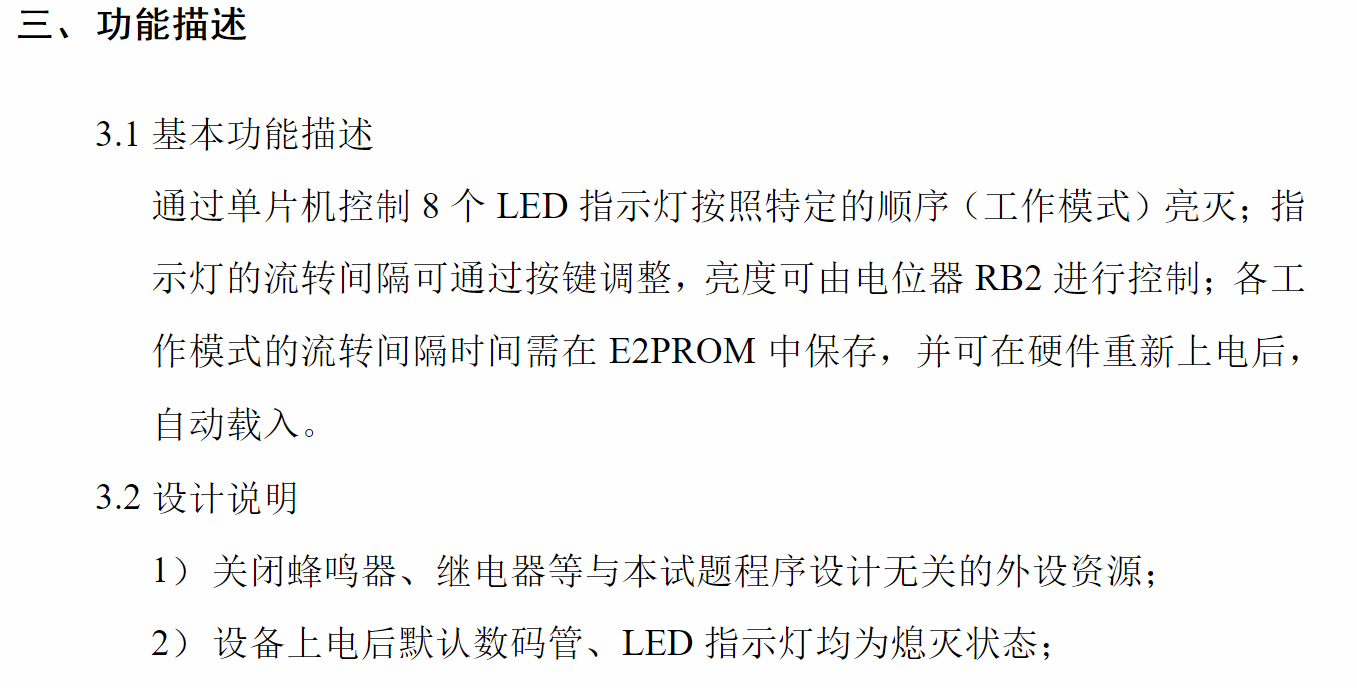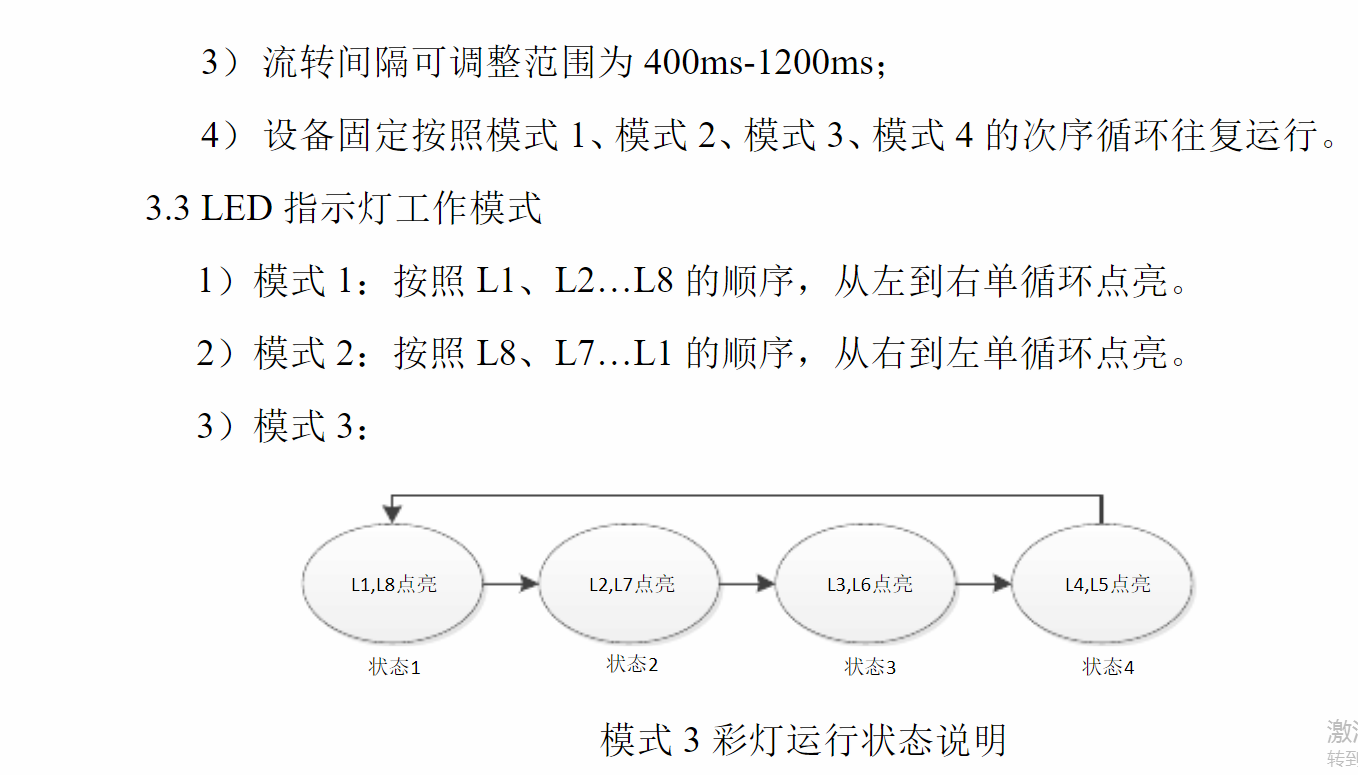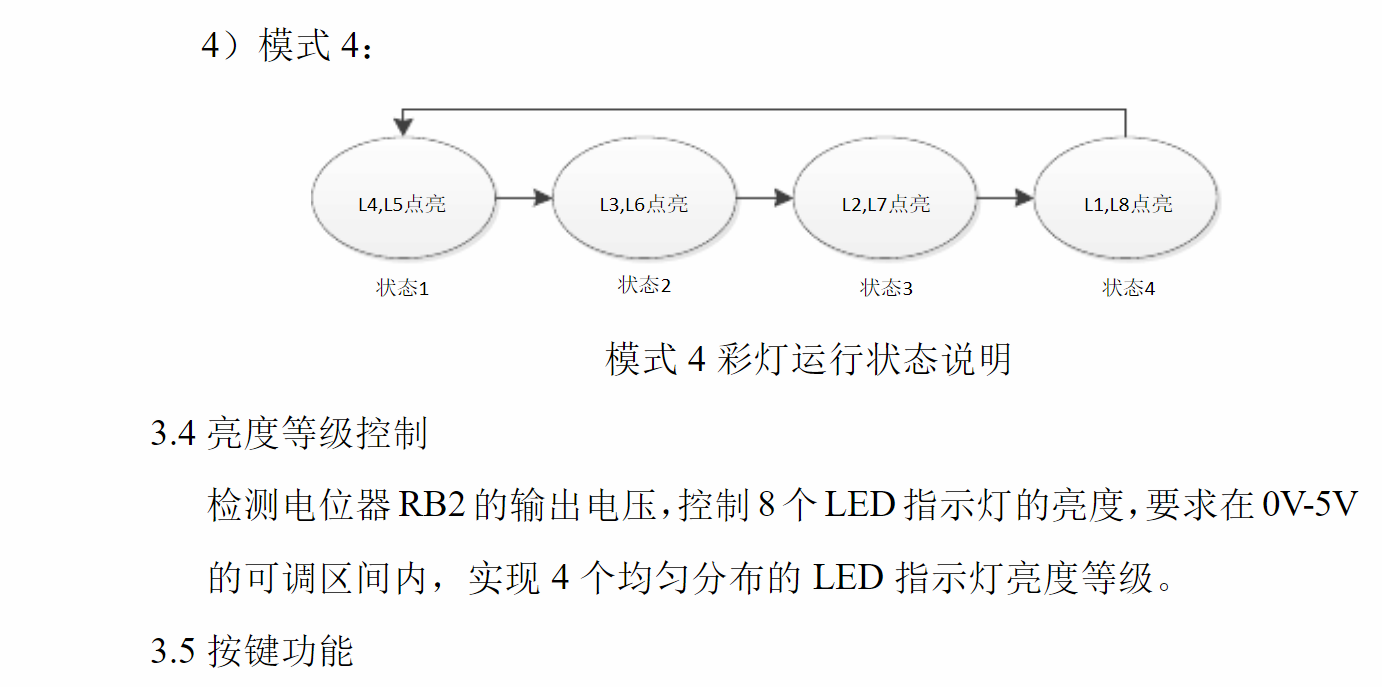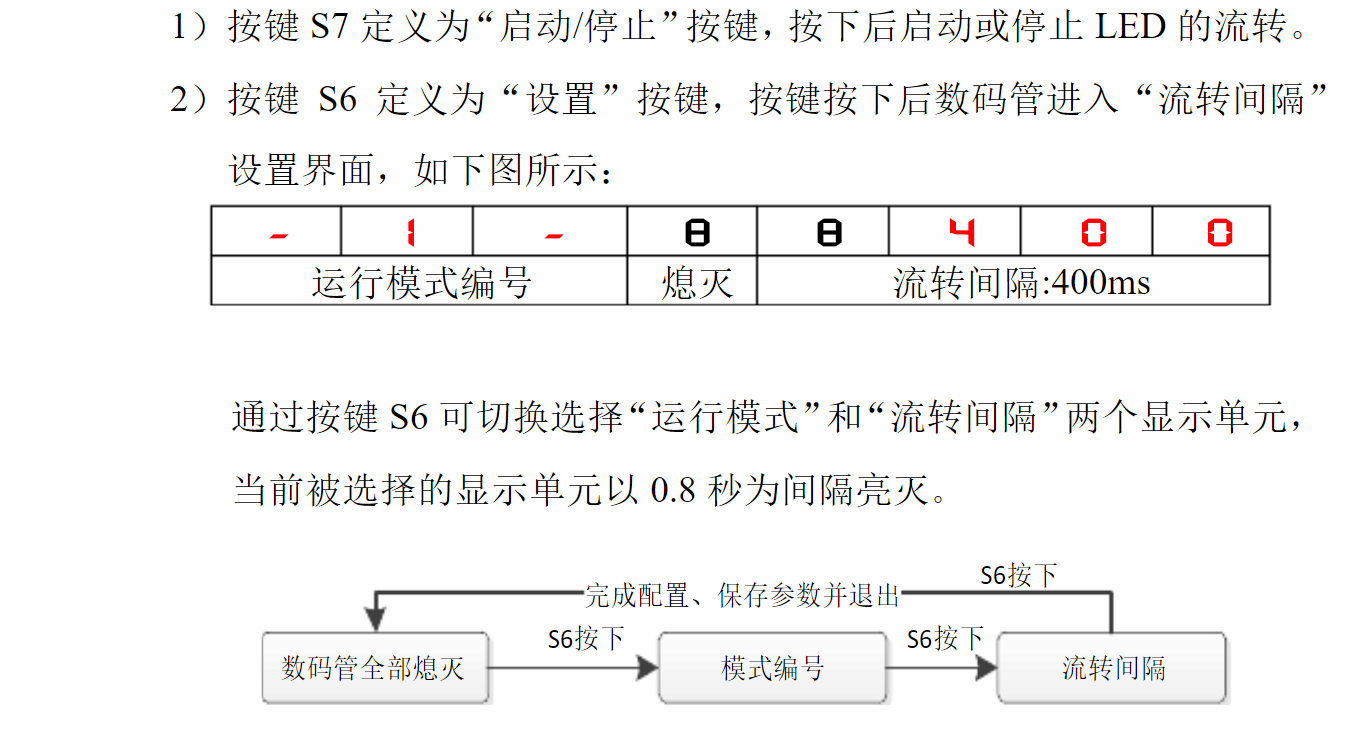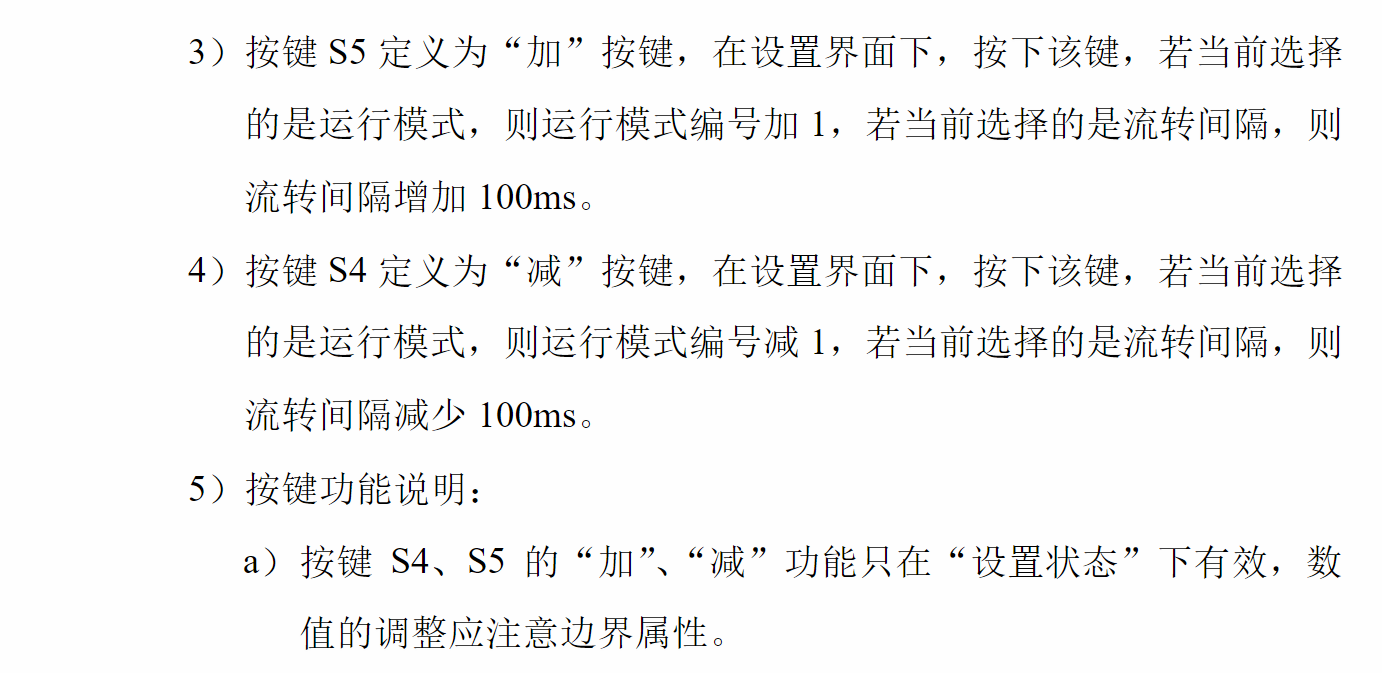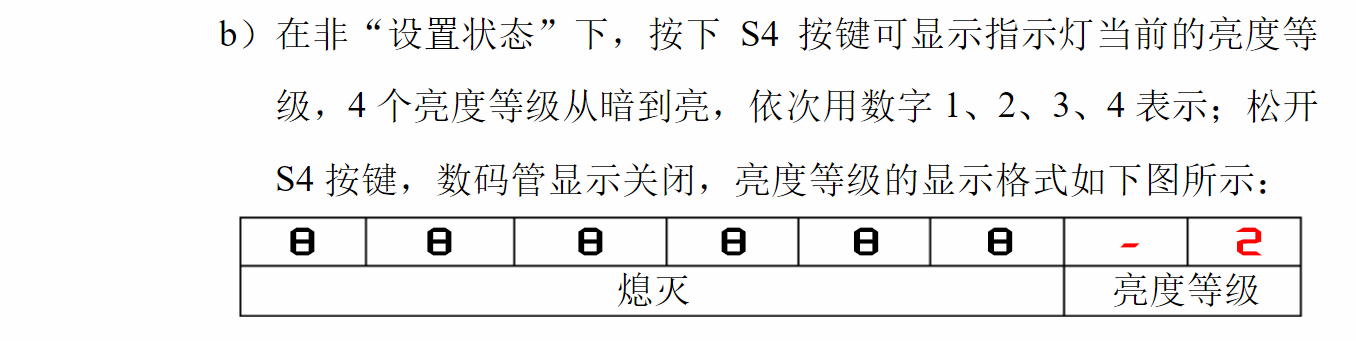个人觉得本题主要考的是定时器，本题如果直接用数码管延时扫描的话会很棘手，之前数码管显示函数我一般都是先位选后段选接着在后面延时个1毫秒，现在这题目用这种方式写好像不太行，正因为不行，我卡在这地方太久了，如果大哥大姐能用这种方法做的话希望你们能教一下我，谢谢！
好了，下面是我刚编完的程序，步数挺多的。。。：
main.c
 #include "STC15F2K60S2.h"
#include "iic.h"
#define uchar unsigned char
#define uint  unsigned int
uchar code tab[]={0xc0,0xf9,0xa4,0xb0,0x99,0x92,0x82,0xf8,0x80,0x90,0xbf,0xff};//数码管段
uchar code mode1_2[]={0xfe,0xfd,0xfb,0xf7,0xef,0xdf,0xbf,0x7f};//模式1.2灯数组
uchar code mode3_4[]={0x7e,0xbd,0xdb,0xe7}; //模式3.4灯数组
uchar yi,er,san,si,wu,liu,qi,ba;//数码管的8个位
uchar jiange=4; //运行间隔.用于显示在数码管上.或赋值给jiange1~jiange4；
uchar modeset=1;//设置运行间隔时的模式
uchar jiange1,jiange2,jiange3,jiange4;
uchar level;    //亮度等级
uchar light; //=PCF8591的3通道
uchar mode=1;//流水灯工作模式
uchar reserve;//保护现场
uchar S6count=0;//0为关数码管状态，1为数码管显示且闪模式位，2为数码管显示且闪运行间隔；
bit S7=0;				//S7按键按下为1表示流水灯启动工作
bit bling=0;			//用于数码管0.8秒闪烁
bit PWM;				//用于亮度
bit flag=0;				//读EEPROM的条件之一
void delayms(uint ms);//1毫秒延时子程序
void keyscan(void);//独立按键扫描
void allinit(void);//关闭继电器蜂鸣器数码管LED灯
void Timer1Init(void);
void Timer0Init(void);
void S6DATAPRO();//S6按键下的一些数据处理
void main(void)
{
//	Write_EEPROM(0x01,4);delayms(10);
//	Write_EEPROM(0x02,4);delayms(10);
//	Write_EEPROM(0x03,4);delayms(10);
//	Write_EEPROM(0x04,4);delayms(10);
jiangge=jiangge1;
allinit();
Timer1Init();
Timer0Init();
EA=1;ET0=1;ET1=1;
while(1)
{
keyscan();
S6DATAPRO();
}
}
void delayms(uint ms)
{
uint i,j;
for(i=ms;i>0;i--)
for(j=845;j>0;j--);
}
void keyscan(void)
{
if(P30==0)
{
//delayms(5);
if(P30==0)
{
if(S7==0){S7=1;TR0 =1;}
else     S7=0;
}
while(!P30);
}
else if(P31==0)
{
delayms(5);
if(P31==0)
{
S6count++;
flag=1;
}
while(!P31);
}
else if(P32==0)
{
//delayms(5);
if(P32==0)
{
flag=1;	//加按键
if(S6count==1)
{
modeset++;
if(modeset==5)modeset=1;
}
else if(S6count==2)
{
jiangge++;
if(jiangge==13)jiangge=12;
}
}
while(!P32);
}
else if(P33==0)
{
//delayms(5);
flag=1;
if(P33==0 && S6count!=0)//减按键
{
if(S6count==1)
{
modeset--;
if(modeset==0)modeset=4;
}
else if(S6count==2)
{
jiangge--;
if(jiangge==3)jiangge=4;
}
}
while(P33==0 && S6count!=0);
if(P33==0 && S6count==0)//显示亮度等级按键
{
yi=er=san=si=wu=liu=11;qi=10;ba=level;
}
}
}
void allinit(void)
{
P2=0xA0;P0=0x00;//关闭凤鸣器继电器
P2=0x80;P0=0xFF;//关闭led灯
P2=0xC0;P0=0xFF;//选择所有数码管
P2=0xFF;P0=0xFF;//关闭所有数码管
}
void S6DATAPRO()
{
if(S6count==0 && P33==1)
{
yi=11;er=11;san=11;si=11;wu=11;liu=11;qi=11;ba=11;
}
else if(bling==1 && S6count==1)
{
yi=10;er=11;san=10;si=11;wu=jiangge/10;liu=jiangge%10;qi=0;ba=0;
}
else if(bling==1 && S6count==2)
{
yi=10;er=modeset;san=10;si=11;wu=11;liu=11;qi=11;ba=11;
}
else if(bling==0 && S6count!=0)
{
yi=10;er=modeset;san=10;si=11;wu=jiangge/10;liu=jiangge%10;qi=0;ba=0;
}
}
void Timer0Init(void)		//100微秒@11.0592MHz
{
AUXR |= 0x80;		//定时器时钟1T模式
TMOD &= 0xF0;		//设置定时器模式
TL0 = 0xAE;		//设置定时初值
TH0 = 0xFB;		//设置定时初值
TF0 = 0;		//清除TF0标志
TR0 = 0;		//定时器0开始计时
}

void Timer1Init(void)		//2毫秒@11.0592MHz
{
AUXR |= 0x40;		//定时器时钟1T模式
TMOD &= 0x0F;		//设置定时器模式
TL1 = 0x9A;		//设置定时初值
TH1 = 0xA9;		//设置定时初值
TF1 = 0;		//清除TF1标志
TR1 = 1;		//定时器1开始计时
}
void TIM0_isr() interrupt 1
{
static uchar PWMcount=0;
static uint  numcount=0;
static uchar number=0;
PWMcount++;numcount++;
reserve=P0;P0=0xff;
if(PWMcount==80)
{
PWMcount=0;
PWM=1;
}
if(level==1 && PWMcount==20)PWM=0;
else if(level==2 && PWMcount==40)PWM=0;
else if(level==3 && PWMcount==60)PWM=0;
else if(level==4)								PWM=1;
if(mode==1 && numcount==jiangge1*1000)
{
numcount=0;
if(S7==1)number++;
if(number==8)
{
number=7;mode=2;
}
}
else if(mode==2 && numcount==jiangge2*1000)
{
numcount=0;
if(S7==1)number--;
if(number==255)
{
number=0;mode=3;
}
}
else if(mode==3 && numcount==jiangge3*1000)
{
numcount=0;
if(S7==1)number++;
if(number==4)
{
number=3;mode=4;
}
}
else if(mode==4 && numcount==jiangge4*1000)
{
numcount=0;
if(S7==1)number--;
if(number==255)
{
number=0;mode=1;
}
}
if(PWM==1)
{
switch(mode)
{
case 1:	P2=0x80;P0=mode1_2[number];break;
case 2: P2=0x80;P0=mode1_2[number];break;
case 3: P2=0x80;P0=mode3_4[number];break;
case 4:	P2=0x80;P0=mode3_4[number];break;
}
}
else if(PWM==0)
{
P2=0x80;P0=0xff;
}
P2=0xff;P0=reserve;
}
void TIM1_isr() interrupt 3
{
static uint shan=0;
static uchar SEG=0;
if(shan==400)
{
shan=0;bling=!bling;
}
{
light=PCF8591(0x03);
if(light<25)level=1;
else if(light<50)level=2;
else if(light<75)level=3;
else if(light<100)level=4;
}
if(S6count==1&& flag==1)
{
switch(modeset)
{
}
}
if(S6count==3)
{

switch(modeset)
{
case 1:	jiangge1=jiangge;Write_EEPROM(0x01,jiangge);break;
case 2:	jiangge2=jiangge;Write_EEPROM(0x02,jiangge);break;
case 3:	jiangge3=jiangge;Write_EEPROM(0x03,jiangge);break;
case 4:	jiangge4=jiangge;Write_EEPROM(0x04,jiangge);break;
}
S6count=0;
}
if(1==SEG)		 { P2=0xC0;P0=0x01;P2=0xFF;P0=0xff;P0=tab[yi]; }
else if(SEG==2){ P2=0xC0;P0=0x02;P2=0xFF;P0=0xff;P0=tab[er]; }
else if(SEG==3){ P2=0xC0;P0=0x04;P2=0xFF;P0=0xff;P0=tab[san];}
else if(SEG==4){ P2=0xC0;P0=0x08;P2=0xFF;P0=0xff;P0=tab[si]; }
else if(SEG==5){ P2=0xC0;P0=0x10;P2=0xFF;P0=0xff;P0=tab[wu]; }
else if(SEG==6){ P2=0xC0;P0=0x20;P2=0xFF;P0=0xff;P0=tab[liu];}
else if(SEG==7){ P2=0xC0;P0=0x40;P2=0xFF;P0=0xff;P0=tab[qi]; }
else if(SEG==8){ P2=0xC0;P0=0x80;P2=0xFF;P0=0xff;P0=tab[ba]; }
else if(SEG==9){ P2=0xC0;P0=0x80;P2=0xFF;P0=0xff;P0=tab; }
if(SEG==9)SEG=0;
}

IIC.c
#include "STC15F2K60S2.h"
#include "iic.h"
#include "intrins.h"

#define somenop {_nop_();_nop_();_nop_();_nop_();_nop_();_nop_();_nop_();_nop_();_nop_();_nop_();_nop_();_nop_();_nop_();_nop_();_nop_();_nop_();_nop_();_nop_();_nop_();_nop_();_nop_();_nop_();_nop_();_nop_();_nop_();_nop_();_nop_();_nop_();_nop_();_nop_();_nop_();_nop_();_nop_();_nop_();_nop_();_nop_();_nop_();_nop_();_nop_();_nop_();}

//总线引脚定义
sbit SDA = P2^1;  /* 数据线 */
sbit SCL = P2^0;  /* 时钟线 */

//总线启动条件
void IIC_Start(void)
{
SDA = 1;
SCL = 1;
somenop;
SDA = 0;
somenop;
SCL = 0;
}

//总线停止条件
void IIC_Stop(void)
{
SDA = 0;
SCL = 1;
somenop;
SDA = 1;
}

//等待应答
bit IIC_WaitAck(void)
{
SDA = 1;
somenop;
SCL = 1;
somenop;
if(SDA)
{
SCL = 0;
IIC_Stop();
return 0;
}
else
{
SCL = 0;
return 1;
}
}

//通过I2C总线发送数据
void IIC_SendByte(unsigned char byt)
{
unsigned char i;
for(i=0;i<8;i++)
{
if(byt&0x80)
{
SDA = 1;
}
else
{
SDA = 0;
}
somenop;
SCL = 1;
byt <<= 1;
somenop;
SCL = 0;
}
}

//从I2C总线上接收数据
unsigned char IIC_RecByte(void)
{
unsigned char da;
unsigned char i;

for(i=0;i<8;i++)
{
SCL = 1;
somenop;
da <<= 1;
if(SDA)
da |= 0x01;
SCL = 0;
somenop;
}
return da;
}
void Write_EEPROM(unsigned char add,unsigned char dat)
{
IIC_Start();
IIC_SendByte(0xA0);
IIC_WaitAck();
IIC_WaitAck();
IIC_SendByte(dat);
IIC_WaitAck();
IIC_Stop();
}
{
unsigned char temp;
IIC_Start();
IIC_SendByte(0xA0);
IIC_WaitAck();
IIC_WaitAck();
IIC_Stop();

IIC_Start();
IIC_SendByte(0xA1);
IIC_WaitAck();
temp=IIC_RecByte();
IIC_Stop();

return temp;
}
{
unsigned char temp;
IIC_Start();
IIC_SendByte(0x90);
IIC_WaitAck();
IIC_WaitAck();
IIC_Stop();

IIC_Start();
IIC_SendByte(0x91);
IIC_WaitAck();
temp=IIC_RecByte();
IIC_Stop();

return temp*0.39;
}

IIC.h
#ifndef _IIC_H
#define _IIC_H

//函数声明
void IIC_Start(void);
void IIC_Stop(void);
void IIC_SendByte(unsigned char byt);
bit IIC_WaitAck(void);
unsigned char IIC_RecByte(void);
void Write_EEPROM(unsigned char add,unsigned char dat);

#endif



展开全文• 这是蓝桥杯官方在单片机比赛时提供的官方资料包，里面包含比赛会用到的相关底层驱动代码，单片机开发板的驱动程序，以及一些辅助开发软件和技术文档，建议有需要的伙伴在比赛前先下载该资料包，提前熟悉官方提供的...
• 往届蓝桥杯省赛题目答案， 第三届：温度监控器。 第四届：自动售水机。 第五届：模拟智能灌溉系统。 第六届：简易温度采集与控制装置。 第七届：模拟风扇控制系统。 第八届：基于单片机的电子钟。
• 1. 本文件夹中提供的驱动代码供参赛选手完成程序设计参考之用， ...使用或调试驱动代码时，选手需根据设定的片内振荡器频率、所使用单片机的机器周期对代码进行调整； 实际使用需要进行相应调整 #include...ds1302驱动程序
• 2019年3月12日更新 在连续多次单字节读取E22PROM时，不需要延时。 搞完所有模块，开始写真题。 1、题目解读 ...看到这个题，大致要知道用到了哪些模块。...在这里我直接把所有代码给贴上来吧，程序可能写的很麻烦，...CT107D 彩灯控制器
• 蓝桥杯单片机组目前使用CT107D实验平台，IAP15F2K61S2芯片 IAP15F2K61S2可以直接当仿真器使用，设置方法： （1）使用STC-ISP v6.85R （2）点击“添加型号与头文件到KEIL中……” （3）将文件定位于.../keil （4）...
• 蓝桥杯总结 // 上电初始化 // 译码器 switch (num) { case 4: P2 = (P2 & 0x1f) | 0x80; break; case 5: P2 = (P2 & 0x1f) | 0xa0; break; case 6: P2 = (P2 & 0x1f) | 0xc0; break; case 7: P2 = ...CT107D
• 我刚开始学完 51单片机的时候，听师兄们说有蓝桥杯这个比赛，所以在寒假开始准备，刚开始很懵逼，拿到板子后都不知道怎么用，怎么入手，当时我也是走了波坑才懂的 所以这里我的建议是上blibli学习小蜜蜂的入门编程...
• 为推动软件开发技术的发展，促进软件专业技术人才培养，向软件行业输送具有创新能力和实践能力的高端人才，提升高校毕业生的就业竞争力，全面推动行业发展及人才...本程序是第七届蓝桥杯单片机设计与开发省赛一等奖程序
• 蓝桥杯 单片机设计 历年决赛真题及答案 在学习蓝桥杯编程时很好的参考，要沉下心分析很精华！
• 蓝桥杯单片机第三届至第八届的代码和题目，这都是我当初练习时写的，有需要的朋友可以下载来看看，不过最好是自己写完或者遇到实在解决不了的问题时才打开来看。
• 蓝桥杯板子一开始拿到手特难用，但是经过一个星期的摸索就会了，感觉蓝桥杯单片机的集成度还是很高的！
• 这是蓝桥杯单片机设计与开发组历届真题和具体代码，有自动售水机、温度监控器、温度采集与控制、模拟智能灌溉等等，全部代码依据蓝桥杯板子写的，下载进去就能用，对于要参加蓝桥杯单片机设计与开发的同学复习非常...
• 蓝桥杯单片机组第二届至第十届省赛赛题及代码 经过实验室多届参赛学生的探索，整合了一套规范的模块化编程风格，代码思路清晰，分享给大家参考。也希望大家从中找出不足加以改正，相互交流学习
• 关于蓝桥杯单片机设计与开发项目模拟试题（客观题部分），其他大多设计模电知识，和c语言编程这里小知识点，主要在于设计题拿分
• 蓝桥杯单片机 赛后资料整理（三）——NE555芯片模块电路原理芯片特性驱动方法代码模板第十届自我总结 本文适用于CT107D竞赛板+IAP15转接板 电路原理 芯片特性 NE555是一种时钟芯片，输出一定频率的脉冲信号。就其...
• 首先看原理图这里的超声波模块主要是介绍的是蓝桥杯板子上的超声波模块,超声波的原理其实很简单的,就是由于单片机一个引脚连接到发射模块,就可以利用这个引脚来发出一定频率的信号,而在,而在发射模块发射出的超声波...物联网
• 蓝桥杯单片机比赛席卷而来，大多数同学资源难觅，因此自己写了一份单片机代码供广大同学参考。第十届省赛试题是将 IAP15F2K61S2 单片机内部振荡器频率设定为 12MHz。其键盘工作模式跳线 J5 配置为 BTN 独立按键模式...
• 这 些 代 码 是 我 当 初 参 加 比 赛 时 练 习 写 的，有 需 要 的 朋 友 可 以 下 载 来 看 看。
• 3.蓝桥杯单片机设计与开发历年真题3.蓝桥杯单片机设计与开发历年真题3.蓝桥杯单片机设计与开发历年真题
• 蓝桥杯单片机省赛记录2019 省赛必考模块 1. LED 2. 数码管显示 3. DS18B20 4. DS1302 5. IIC、AD转换 省赛历年必做真题 1. 第四届（模拟智能农田灌溉系统） 2. 第五届（温度检测与控制装置） 时钟 IIC AD 独立按键 ...单片机PWM调制
• **第十一届蓝桥杯 单片机设计与开发 省赛** 在昨天举办了第一波11届蓝桥杯比赛，第2波大概在10月份左右，看了看比赛题目，这次题目对于我来说，程序设计题简单，客观题全靠蒙！ 本次比赛涉及: 1.数码管显示 2.矩阵...
• 蓝桥杯单片机不是时，官方会提供给参赛者的数据包，分享出来，供大家参考
• 第十一届蓝桥杯单片机省赛2020年四月份模拟试题 ** i2c.c #include "i2c.h" #include "intrins.h" #include "STC15F2K60S2.H" #define DELAY_TIME 5 #define SlaveAddrW 0xA0 #define SlaveAddrR 0xA1 //总线...
• 第十届蓝桥杯单片机设计与开发项目省赛-客观试题dvsdv...